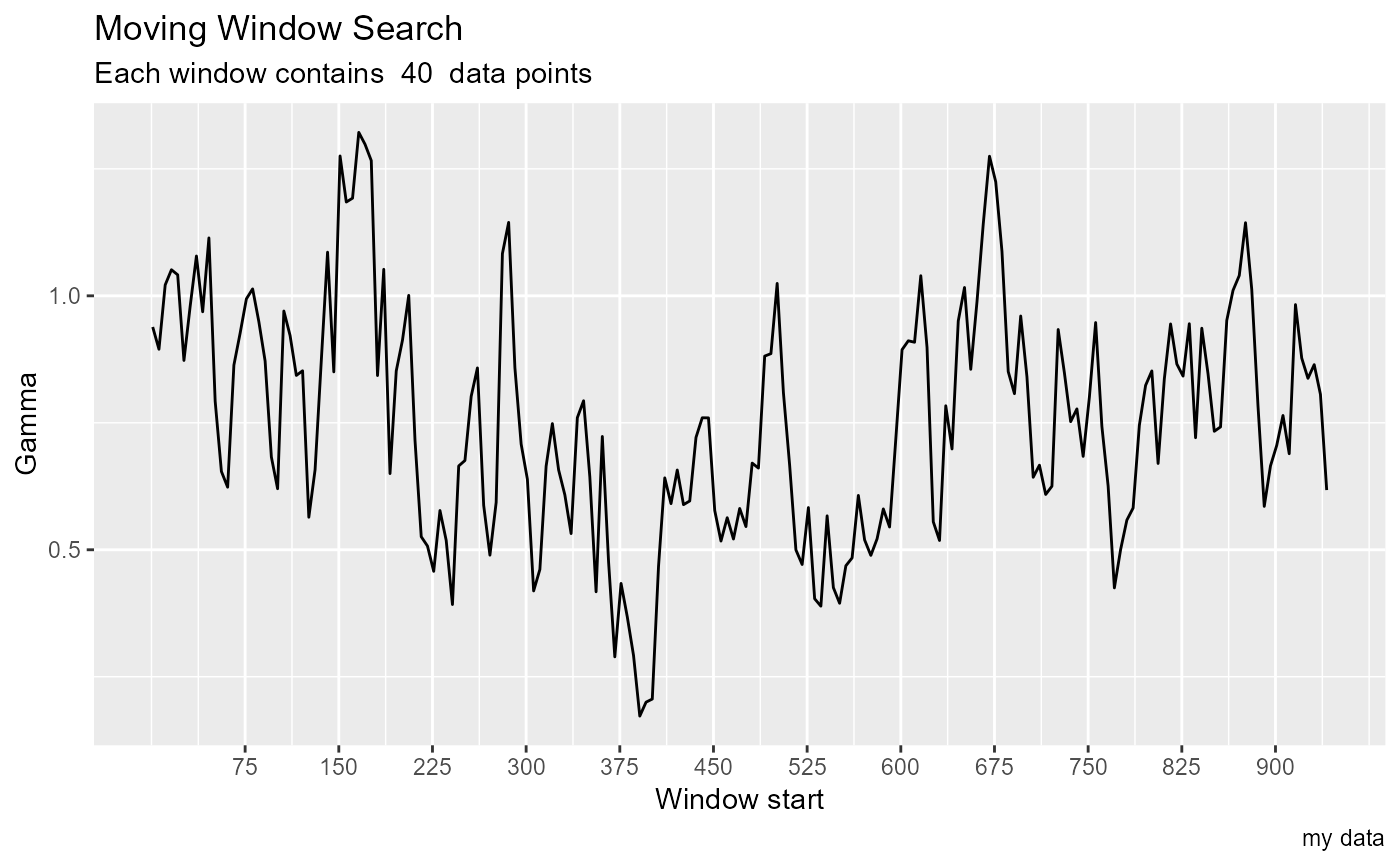Calculate Gamma values for a window moving through the data.

## Usage

moving_window_search(
predictors,
target,
window_size = 40,
by = 1,
plot = TRUE,
caption = "",
show = "Gamma"
)

## Arguments

predictors

A Numeric vector or matrix whose columns are proposed inputs to a predictive function

target

A Numeric vector, the output variable that is to be predicted

window_size

Integer width of the window that will move through the data

by

The increment between successive window starts

plot

Logical, set this to FALSE if you don't want the plot

caption

Character string, caption for plot

show

Character string, if it equals "vratio", vratios will be plotted, otherwise Gamma is plotted

## Value

An invisible data frame containing starting and ending positions of each window with its associated gamma

## Details

This is used for data sets that are ordered on one or more dimension, such as time series or spatial data. The search slides a window across the data set, calculating gamma for the data at each step. A change in causal dynamics will appear as a spike in gamma when the causal discontinuity is in the window.

## Examples

he <- embed(henon_x, 13)
t <- he[ , 1]
p <- he[ ,2:13]
moving_window_search(p, t, by = 5, caption = "my data")